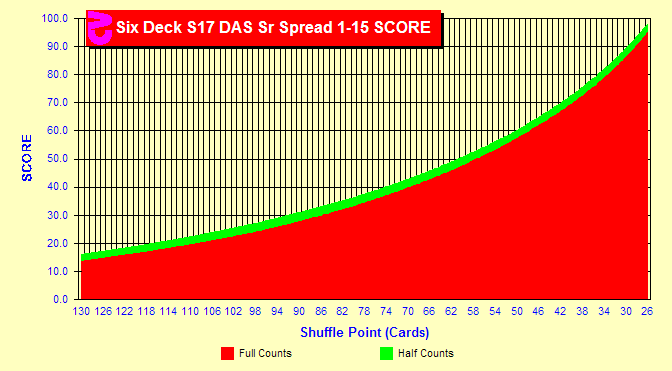# Blackjack Betting by Half Counts

###Should I bet by half counts?

Most card counters determine the best bets to make at each count before sitting at the table. We know that we should bet more at higher counts. For example you might bet 1 unit at counts of zero and below, 2 units at a count of +1, 5 units at a count of +2, etc. Can we improve on this by creating a table based on half counts? That is, can we improve our SCORE by betting 3 units at a count of +1.5? As in most areas of Blackjack, the answer is — it depends.

In this chart we show the SCOREs for all reasonable penetrations in a six-deck game using Hi-Lo. The red area represents the SCOREs using bets based only on integer counts. The green area is the gain realized in a more complex table using different bets for each half count. The difference is so slight that you can barely see a hint of green above the red area. There is no appreciable gain. But this doesn't tell the whole story.

###What about Zen?

In the second chart we look at the same process, but using the 1998 Zen strategy. Using this strategy we now see a green area. In fact, at 4.5/6 deck penetration in the middle of the chart; the gain from using half-counts is 9.3%. What happened?

The Hi-Lo strategy calculates true counts by dividing by the number of remaining decks. The 1998 Zen strategy calculates TCs by dividing by the remaining quarter decks. This is a much higher divisor; so you end up with a much smaller range of true counts. With a smaller range of counts, there is less resolution and this means less accuracy. You can gain back most of this accuracy by betting according to half-counts instead of integer counts when using Zen.

Note: unbalanced strategies like KO, KISS and Red7 have a very large range of counts and do not need betting by half-counts.

### Sim details

• Six decks, S17, DAS, LS 1 player, Hi-Lo, truncate, Sweet 16 & Fab 4 indexes, half-deck resolution, 26-130 cards penetration
• Six decks, S17, DAS, LS 1 player, 1998 Zen, floor, Sweet 16 & Fab 4 indexes, half-deck resolution, 26-130 cards penetration
• Optimal betting by full and half counts
• Two billion rounds each